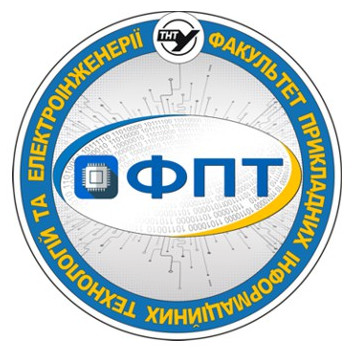## Syllabus

Select major*Ternopil Ivan Puluj National Technical University

Факультет прикладних інформаційних технологій та електроінженерії

Кафедра біотехнічних систем

Metrology

syllabus

 Major 163 - Біомедична інженерія (бакалавр) Field of knowledge 16 Хімічна та біоінженерія Academic degree bachelor's
Course
Course type required
special education
Study start course 2
Semesters 4
Form of education full-time
Study hours structure
 36 – lectures 18 – practical classes 18 – laboratory classes
Amount of hours for individual work 88
ECTS credits 6
Form of final examination exam
Lecturer
 Academic degree Cand. Sc. Academic title Assoc. Prof. Full name Тимків Павло Олександрович
Prerequirements (prerequisite courses)
 Higher Mathematics, Physics
Course goals and learning objectives
 Purpose: the acquisition of theoretical knowledge and practical skills by students in the organization of measurements of electrical, magnetic and non-cellular quantities and the evaluation of their accuracy. Objectives: studying the general provisions of the theory of accuracy, methods for assessing the accuracy of the results of measurements of the values of physical quantities, as well as the basic principles of construction and application of measuring instruments.
Course description
 Lectures 1. Subject and content of metrology. Subject, methods, means and main directions of metrology. Experimental methods of cognition. 2. Physical value is the basic concept of metrology. Physical value (PV). Value, dimension and unit of PV. Basic and additional units of the International System of Units SI. Systematization of PV. 3. Measurement of physical quantities. Classification of measurements. Basic measurement equation. Significant signs of measurement and algorithms for their implementation. Direct and indirect (mediated, cumulative, compatible) measurements. 4. The most significant components of the measurement experiment. Measurement conditions. Methods and methods of measurement. Algorithm for measuring the operation. The main components of the measuring expriment. 5. Measuring devices. Metrological characteristics. Measure. Measurement transformation. Scalable and numerical measurement transformations. Comparison of PV. Measuring transducer. 6. Measuring tools. Principle of operation, measuring circle and types of measuring instrument circuits (IC). Structural scheme and types of transformation. Generalized scheme of information-measuring system. 7. Measurement errors. True and true value of PV. Absolute and relative errors of measurements. Classification of measurement errors. Measurement accuracy. Excessive error Miss. Correctness, convergence and reproduction of measurements. 8. Measurement Absolute, relative and aggregated errors of measuring instruments (MI). The main and additional errors of the MI. MI parameters. The show, countdown, became an MI, the price scale of the division. Range of impressions, boundaries and range of measurements. Class of accuracy. 9. Random mistakes. The nature of the occurrence of random errors. Gauss distribution. Uniform distribution law. Basic numerical characteristics. 10. Processing of the results of direct and indirect measurements. Rounding rules and form of presentation of measurement results. Rounding error. Detection of failures among measurement results. Estimates of errors of direct single and multiple measurements. 11. Means of Measurement Properties. MI classification. Basic static metrological characteristics of MI. The main dynamic metrological characteristics of the MI. 12. Digital and analogue electronic devices. The main methods (sequential account, sequential approximation and parallel method) transform noncontiguous measuring quantities into numerical codes. Principles of construction of the chronometer, time-pulse voltmeter, frequency meter, centimeter and ohmmeter. 13. Measurement signal signals. Basic concepts. Types of measuring signals. Mathematical models of measuring signals. 14. Ensuring the unity of measurements. Fundamentals of Metrological Measurement Assurance. Some provisions of the metrological certification of the MI. Verification MI. Practical classes 1. Practical work №1 Removing systematic errors 2. Practical work №2 Detection of failures among measurement results. 3. Practical work №3 Rounding rules and form of presentation of measurement results. 4. Practical work №4 Work out the results of direct single and multiple measurements. 5. Practical work №5 Working out the results of mediated measurements. 6. Practical work №6 Working out the results of combined and compatible measurements. Laboratory classes Laboratory work №1 Measuring generator. Laboratory work №2 Digital voltmeter. Laboratory work №3 Electronic oscilloscope. Laboratory work №4 Measurement of the amplitudes of electrical signals and time intervals using an oscilloscope. Laboratory work №5 Measurement of time intervals, frequencies using a frequency meter.
Assessment criteria
 Module 1 – 40 points: 10 points – test, 15 points – lab works. 15 points – pract. works. Module 2 – 35 points: 10 points – test, 10 points – lab works. 15 points – pract. works. Form of final control – examination – max 25 points